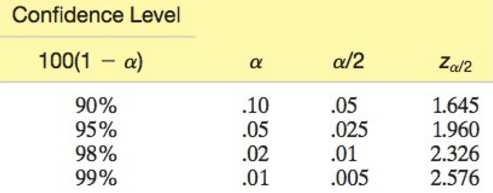# Large & Small Sample Confidence Interval for Mean – Theory

Confidence Interval for Mean, µ.

Large-sample 100*(1-α)% Confidence interval using Z-distributionwhere Zα/2 is the z-value corresponding to an area α/2 in the tail of the standard normal distribution, and σx ̅ is the standard deviation of the sampling distribution, σ is the population standard deviation, and s is the standard deviation of the sample.

Conditions required for a valid large-sample confidence interval for µ

• A random sample is selected from the target population
• The sample size n is large (n > 30). Due to the Central Limit Theorem, this condition insures that the sampling distribution is approximately normal and that s will be a good estimator of σ.

Commonly used values of Zα/2Small Samples

With small samples, n < 30, use of the standard normal z as a test statistic is problematic for making an inference about the mean µ.

• The Central Limit Theorem only applies for large samples; therefore, we cannot assume the sampling distribution of x-bar is approximately normal.
• The population standard deviation, σ, is almost never known except for academic problems.

Instead of the standard normal statistic, use the t-statistic:where s is the sample standard deviation.

The t-distribution has a mean of 0 like the z-distribution, but its shape depends on the sample size n. In the t-distribution tables, we use the degrees of freedom, n – 1, and α to find the value of the t-statistic, tα.

The reason we set 30 as the determinant of whether to use the z or the t distribution, is that as n increases, the difference between the t-statistic and are essentially identical.

To form the confidence interval for a small sample from a normal distribution, use the following formula:Note: if σ is known and the target population has a relative frequency distribution that is approximately normal, you can use the z-statistic to form the confidence interval.

This site uses Akismet to reduce spam. Learn how your comment data is processed.